# 1st PUC Computer Science Question Bank Chapter 10 Control Statements

## Karnataka 1st PUC Computer Science Question Bank Chapter 10 Control Statements

### 1st PUC Computer Science Control Statements One Mark Questions and Answers

Question 1.
What is a statement?
Statements are the instructions given to the computer to perform specific of actions. Action may be in the form of data movement, decision making, etc.

Question 2.
What are control statements?
Control statements are elements in the program that can control and alter the sequence of flow of constructions in any program execution.

Question 3.
Write the classification of control statements.
The control statements are classified as

1. Selection statements
2. Iterative statements and
3. Jump statements.

Question 4.
What is meant by a compound statement?
A compound statement is a grouping of statements enclosed within a pair of braces ({ }), in which each individual statement ends with a semi-colon.

Question 5.
What are selection statements?
The selection statements are used to execute certain block of statements after evaluating the condition.

Question 6.
What is a block?
A group of statements separated by semicolons and grouped together and enclosed within brackets marked with { } is called a block.Question 7.
What is the purpose of ‘if’ statement?
The ‘if’ statement is used to decide on whether a statement or a block should be executed or not.

Question 8.
What is the other name of ‘if’ statement?
The other name of ‘if’ statement is one-way branching statement.

Question 9.
Give the possible output of condition evaluation.
The possible output of condition evaluation is either TRUE or FALSE.

Question 10.
What is the numerical equivalent of TRUE or FALSE?
The numerical equivalent of TRUE is 1 and FALSE is 0.

Question 11.
What is the other name of ‘if-else’ statement?
The other name of ‘if-else’ statement is two-way branching.

Question 12.
When is ‘if-else’ statement used?
‘If else’ statement is a conditional branching statement used to execute a set of codes when the condition is true, otherwise executes a different set of the codes in the “else” part.

Question 13.
What is an ‘if-else-if’ statement?
The ‘if-else if’ statement is an extension of the “if-else” conditional branching statement. When the expression in the “if’ condition is “false” another “if-else” construct is used to execute a set statement based on an expression.

Question 14.
What is ‘nested if’?
An if statement nestling inside another ‘if’ statement is termed as ‘a nested if’ statement.

Question 15.
Give the name of multi-branch selection statement.
The multi-branch selection statement is called as ‘switch-case’ statement.Question 16.
Give the purpose of the switch statement.
The ‘switch’ statement is used in C++ for testing whether a variable is equal to any one of a set of values.

Question 17.
Define the term looping.
Looping means execution of a statement or block of statements repeatedly until the condition is true.

Question 18.
Why is ‘while’ loop called a pre-tested looping statement?
The ‘while’ loop evaluates the condition in the beginning itself, to select a suitable statement for execution repeatedly. Therefore it is called a pre-tested looping statement.

Question 19.
What is post-tested looping statement?
The loop evaluates the condition at the end of the looping structure and selects a statement for execution repeatedly. Therefore it is called a post-tested looping statement.

Question 20.
Name the post-tested looping statement.
The ‘do-while’ statement is a post-tested looping statement.

Question 21.
Which structure is called a fixed-execution looping statement?
The ‘for’ looping statement is called a fixed-execution looping statement.

Question 22.
When is ‘for’ used?
If the programmer knows the exact number of repetitions to be carried out among the set of statements then ‘for’ conditional statement is used in a program.

Question 23.
What is nesting of loop?
If the loop appears inside the body of another loop it is called a nested loop.

Question 24.
What is the purpose of ‘break’ statement?
The ‘break’ statement can be used to terminate a repeated structure (loops) such as ‘while’, do-while ‘and’ ‘for’ and multi-branching statements like ‘switch’.

Question 25.
What is the use of ‘goto’ statement?
The ‘goto’ statement transfers/jump control from one part of the program to some other part.

Question 26.
What is the function of ‘continue’ statement?
The ‘continue’ statement is used in loops and causes a program to skip the rest of the body of the loop.Question 27.
Why is the ‘exit() library’ function used?
The execution of a program can be stopped at any point with ‘exit ( )’ and a status code can be informed to the calling program.

### 1st PUC Computer Science Control Statements Two/Three Marks Questions and Answers

Question 1.
What are control statements? How are they classified?
Control statements are elements in the program that control the flow of program execution. Control statements are classified as,

1. Selection statements
2. Iterative statements
3. Jump statements

Question 2.
What are selection statements? Give the different types of selection statements.
Selection statements are used to execute certain block of statements by evaluating the conditions.
The different selection statements are

1. ‘if’ statement
2. ‘if-else’ statement
3. ‘if-else-if’ (nested) statement
4. ‘switch’ statement

Question 3.
Give the syntax of ‘if’ statement.
The syntax of if statement is
if (condition)
{
statement 1;
statement 2;
statement n;
}Question 4.
Write the flowchart representation of ‘if’ statement.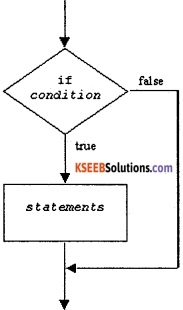Question 5.
Write the syntax of ‘if-else’ statement.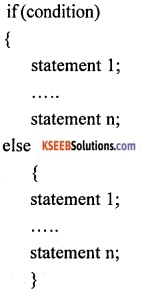Question 6.
Write the flowchart representation of ‘if-else’ statement.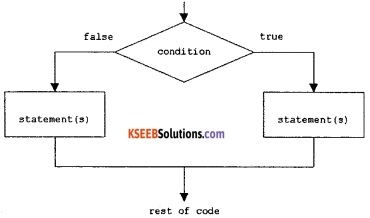Question 7.
Write the syntax of if-else-if statement.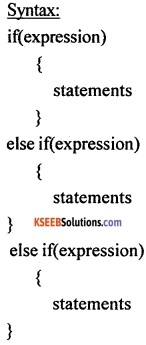Question 8.
Write the flowchart representation of ‘if-else-if’ conditional structure.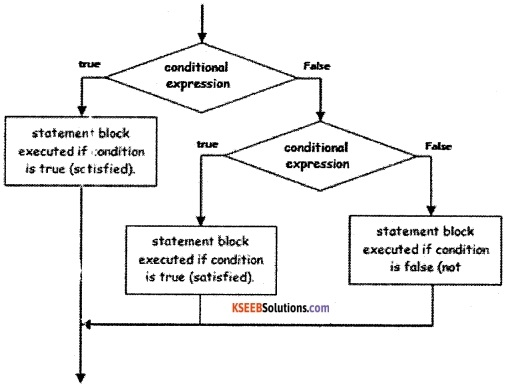Question 9.
Write the syntax of‘switch-case’ statement.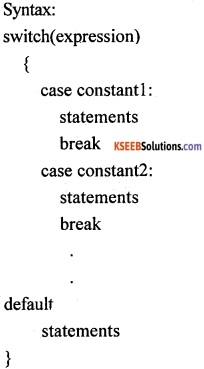Question 10.
Write the comparison between ‘if-else’ and ‘switch-case’ statement.
The ‘if-else’ statements permit two-way branching, whereas ‘switch’ statement permits multiple branching.

Question 11.
Write the syntax of ‘while’ loop.
Syntax of ‘while’ loop:
while(condition)
{
statement(s);
}Question 12.
Write the syntax of ‘do-while’ loop.
Syntax of ‘do-while’ loop
do
{
statements;
} while (condition);

Question 13.
Write the flowchart of representation of while loop.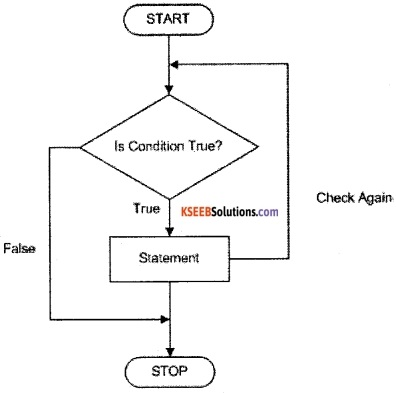Question 14.
Write the flowchart representation of ‘do-while’ loop.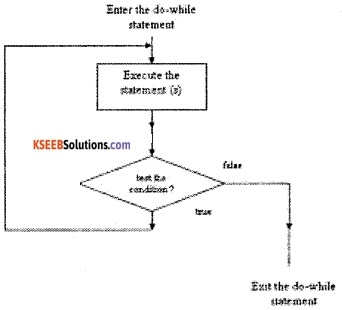Question 15.
Give the difference between ‘break’ and ‘exit()’ statements.
The ‘break’ statement can be used to terminate a repeated structure (loops) such as ‘while’, do-while and for and multi-branching statements like ‘switch’.
The execution of a program can be stopped at any point with ‘exit ( )’.

Question 16.
Give the difference between ‘goto’ statement and ‘continue’ statement.
The ‘goto’ statement transfers/jump control from one part of the program some other part. The ‘continue’ statement is used in loops and causes a program to skip the rest of the body of the loop.

### 1st PUC Computer Science Control Statements Five Marks Questions and Answers

Question 1.
Explain the working of ‘ if’ statement and ‘if-else’ statement with examples.
Example for ‘if’ statement: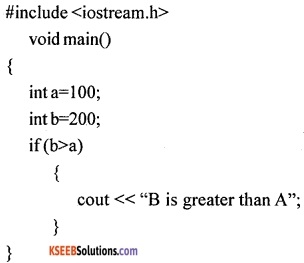Result:
B is greater than A
In the above example the condition in the if statement returns a true value and so the text “B is greater than A” is displayed.
Example for ‘if else’ statement: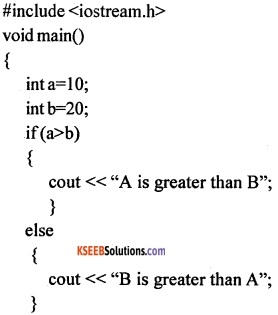Result:
B is greater than A.
In the above example, the “if’ condition is false so the code in the “else” part is executed.Question 2.
Explain the working of if-else if statement with a suitable example.
The ‘if-else if’ statement is an extension of the “if-else” conditional branching statement. When the expression in the “if’ condition is “false” another “if-else” construct is used to execute a set of statements based on the expression.
Syntax:
if(expression)
{ statements }
else if(expression)
{ statements }
else if(expression)
{ statements }
Example: for ‘if else if’ statement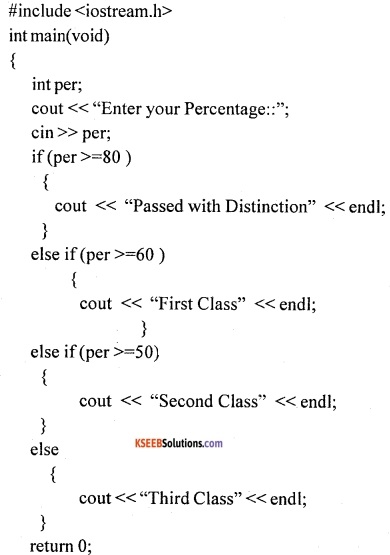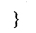Result:
First Class
In the above example, the ‘if else if’ statement is used to check multiple conditions based on the percentage of marks got, as input. The user entered percentage as 60, then first condition evaluated and returned with false. This resulted in checking for second if condition and resulted in true which give the print “first class”.

Question 3.
Write a short note on ‘switch-case’ statement.
A ‘switch’ statement allows a variable to be tested for equality against a list of values. Each value is called a case, and the variable being switched on is checked for each case.
Syntax:
The syntax for a switch statement:
switch(expression)
{
case constant-expression : statement(s);
break; //optional
case constant-expression : statement(s);
break; //optional
default                              : statement(s);                 //Optional
}
The following rules apply to a switch statement:

1. The expression used in a ‘switch’ statement must have an integer or enumerated type.
2. We can have any number of case statements within a ‘switch’. Each case is followed by the value to be compared to, and a colon.
3. The constant-expression for a case must be the same data type as the variable in the ‘switch’,
and it must be a constant or a literal.
4. When the variable being switched on is equal to a case, the statements following that case will execute until a ‘break’ statement is reached.
5. When a ‘break’ statement is reached, the ‘switch’ terminates, and the flow of control jumps to the next line following the ‘ switch ’ statement.
6. Not every case needs to contain a break. If no break appears, the flow of control will fall through to subsequent cases until a ‘break’ is reached.
7. If no conditions match, then the code under the default statement is executed.
8. The default case is used for performing a task when none of the cases is ‘true’.
9. No break is needed in the default case.Question 4.
Explain ‘switch-case’ statement with a suitable program example.
‘ Switch’ statement compares the value of an expression against a list of integers or character constants. The list of constants are listed using the “case” statement along with a “break” statement to end the execution.
Example: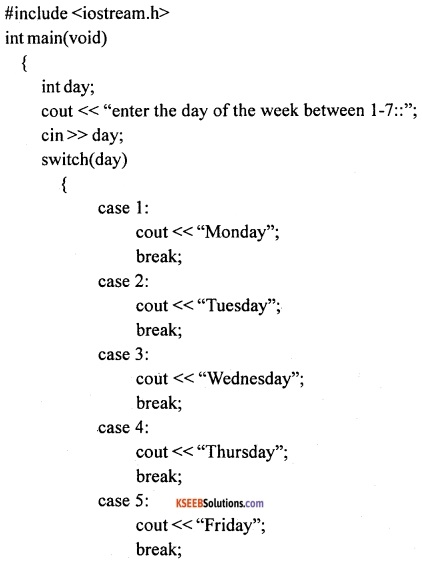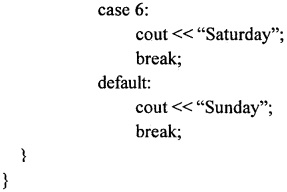Result:
Enter the day of the week between 1-7::7
Sunday
In the above Control Structure example, the “switch” statement is used to find the day of the week from the integer input got from the user. The value present in the day is compared for equality with constants written in the word case. Since no equality is achieved in the above example (from 1 to 6), when 7 is entered default is selected and gives “Sunday” as a result.

Question 5.
Explain ‘while’ loop structure with an example.
A ‘while’ loop statement repeatedly executes a statement or sequence of statements written in the flower brackets as long as a given condition returns the value ‘true’.
Syntax:
The syntax of a ‘while’ loop in C++ is:
while(condition)
{
statement (s) ;
}
Here the condition may be any expression, and for true is any non zero value. The loop iterates while the condition is true. When the condition becomes false, program control passes to the line immediately following the loop. During the first attempt, when the condition is tested and the result is false, the loop body will be skipped and the first statement after the ‘while’ loop will be executed.
Example: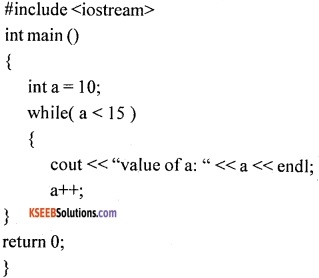When the above code is executed, it produces the following result:
value of a: 10
value of a: 11
value of a: 12
value of a: 13
value of a: 14Question 6.
Explain the working of ‘for’ loop structure.
A ‘for’ loop is a repetition control structure, that allows you to efficiently write a loop that needs to execute a specific number of times.
Syntax:
The syntax of a ‘for’ loop in C++ is:
for (initialization; condition; increment/decrement)
{
statement(s);
}
The working of a ‘for’ loop:

1. The initialization step is executed first, and only once in the beginning. It is used to declare and initialize loop control variables.
2. Next, the condition is evaluated. If it is true, the body of the loop is executed. If it is false, the body of the loop does not execute and the flow of control jumps to the next statement just after the ‘for’ loop.
3. After the body of the ‘for’ loop executes, the flow of control jumps back up to the increment/ decrement statement and update any ‘loop’ control variables.
4. Then the condition is evaluated again. If it is true, the ‘ loop’ executes and the process repeats itself (body of ‘loop’, then increment step, and then again condition). After the condition becomes false, the ‘for’ loop terminates.

Example: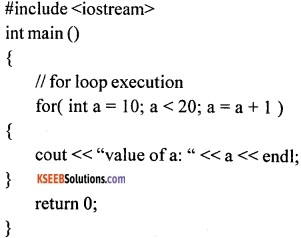When the above code is compiled and executed, it produces following result:
value of a: 10
value of a: 11
value of a: 12
value of a: 13
value of a: 14
value of a: 15